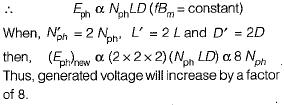Courses

# Basic Concept Of Rotating Electrical Machines Of Electrical Machines - 1

## 10 Questions MCQ Test Topicwise Question Bank for GATE Electrical Engineering | Basic Concept Of Rotating Electrical Machines Of Electrical Machines - 1

Description
This mock test of Basic Concept Of Rotating Electrical Machines Of Electrical Machines - 1 for Electrical Engineering (EE) helps you for every Electrical Engineering (EE) entrance exam. This contains 10 Multiple Choice Questions for Electrical Engineering (EE) Basic Concept Of Rotating Electrical Machines Of Electrical Machines - 1 (mcq) to study with solutions a complete question bank. The solved questions answers in this Basic Concept Of Rotating Electrical Machines Of Electrical Machines - 1 quiz give you a good mix of easy questions and tough questions. Electrical Engineering (EE) students definitely take this Basic Concept Of Rotating Electrical Machines Of Electrical Machines - 1 exercise for a better result in the exam. You can find other Basic Concept Of Rotating Electrical Machines Of Electrical Machines - 1 extra questions, long questions & short questions for Electrical Engineering (EE) on EduRev as well by searching above.
QUESTION: 1

### A linear magnetic circuit has flux linkages of 1.2 Wb turns when a current of 10 A flows through its coil. The energy stored in the magnetic field of this coil, in Joules is

Solution: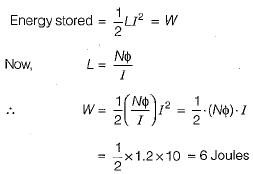QUESTION: 2

Solution:
QUESTION: 3

### Singly-and doubly-excited magnetic systems are respectively

Solution:

In synchronous-motor, dc excitation is given to rotor for flux generation while it’s stator is connected is 3-φ supply. Therefore, it has doubly- excited system . Reluctance motor is an excitation-less motor ie. it has singly-excited magnetic system.

QUESTION: 4

A rotating electromechanical energy conversion device has uniform air-gap. If δ is the space angle between the axis of stator field and rotor field, then the average torque developed is proportional to (a and b are constants)

Solution:

Average torque developed is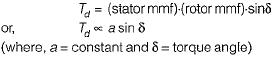QUESTION: 5

Armature winding is one in which working

Solution:
QUESTION: 6

In ac rotating machines, the generated or speed emf

Solution:

In ac rotating machines like synchronous generators/motor/induction motors, working flux (φ) leads generated emf by 900. However, in static device like a transformer working flux (φ) lags the applied voltage by 900.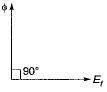QUESTION: 7

A 3-phase, 4-pole alternator has 48 stator slots carrying a 3-phase distributed winding. Each coil of the winding is short chorded by one slot pitch. The belt-factor of the winding is given by

Solution:

m = number of slots/pole /phase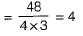Number of slots /pole = 48/4 = 12
∴ slot-pitch angle,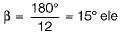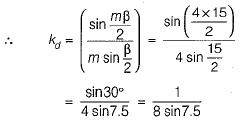QUESTION: 8

Consider the following statements about the chorded coils:
1. Chorded coil leads to reduction in harmonics.
2. In chorded coils, coil span may be less or more than 180° electrical.
3. Chorded coils require more conductors material.
4. Chorded coils reduce the magnitude of output voltage.
5. Chorded coils give better generated emf waveform.
6. In chorded coils, coil span is less than 180° electrical.
From these, the correct statements are:

Solution:
QUESTION: 9

For eliminating 3rd harmonic from the phase emf generated in an alternator, the coil span in terms of full-pitch would be

Solution:

For eliminating nth harmonic,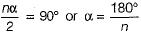∴ Coil span of short-pitched winding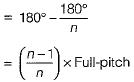For eliminating 3rd harmonic, coil-span
= 2/3 x Full-pitch

QUESTION: 10

If the dimensions of all the parts of a synchronous generator, and the number of field and armature turns are doubled, then the generated voltage will change by a factor of

Solution:

Emf induced per phase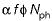Here,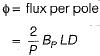( L = Axial length of armature and D = diameter)